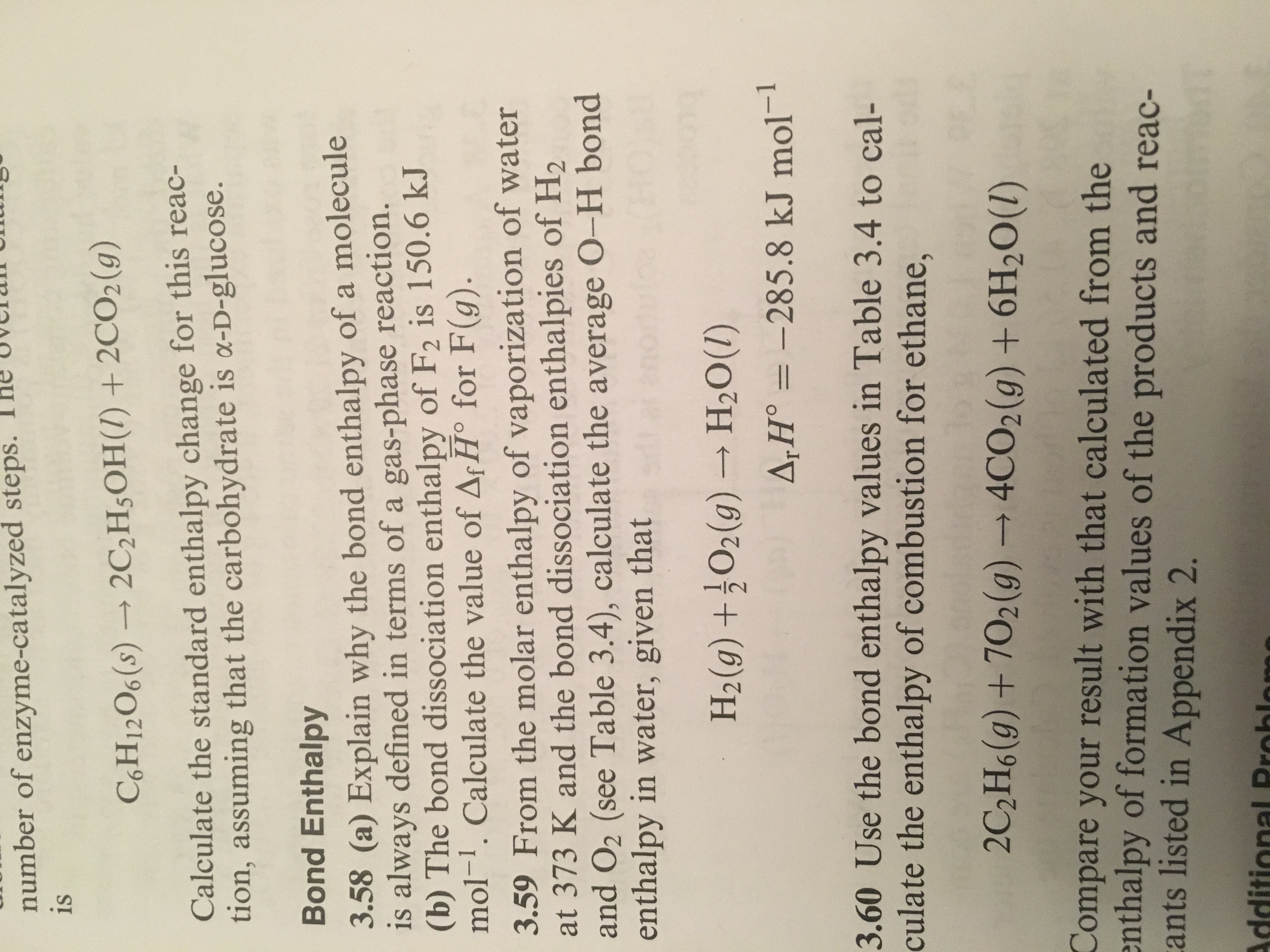# steps. The uvelan U number of enzyme-catalyzed is C6H1206 (s) → 2C2H3OH(I) + 2CO2(g) Calculate the standard enthalpy change for this reac- tion, assuming that the carbohydrate is a-D-glucose. Bond Enthalpy 3.58 (a) Explain why the bond enthalpy of a molecule is always defined in terms of a gas-phase reaction. (b) The bond dissociation enthalpy of F2 is 150.6 kJ mol-1. Calculate the value of ArHo for F(g) 3.59 From the molar enthalpy of vaporization of water at 373 K and the bond dissociation enthalpies of H2 and O2 (see Table 3.4), calculate the average O-H bond enthalpy in water, given that H2(0) +02(9) H2Ou) 285.8 kJ mol-1 3.60 Use the bond enthalpy values in Table 3.4 to cal- culate the enthalpy of combustion for ethane, 2C2H6(g) + 702 (g) → 4CO2(g) + 6H20(1) Compare your result with that calculated from the nthalpy of formation values of the products and reac- ants listed in Appendix 2.

Question

3.58help_outlineImage Transcriptionclosesteps. The uvelan U number of enzyme-catalyzed is C6H1206 (s) → 2C2H3OH(I) + 2CO2(g) Calculate the standard enthalpy change for this reac- tion, assuming that the carbohydrate is a-D-glucose. Bond Enthalpy 3.58 (a) Explain why the bond enthalpy of a molecule is always defined in terms of a gas-phase reaction. (b) The bond dissociation enthalpy of F2 is 150.6 kJ mol-1. Calculate the value of ArHo for F(g) 3.59 From the molar enthalpy of vaporization of water at 373 K and the bond dissociation enthalpies of H2 and O2 (see Table 3.4), calculate the average O-H bond enthalpy in water, given that H2(0) +02(9) H2Ou) 285.8 kJ mol-1 3.60 Use the bond enthalpy values in Table 3.4 to cal- culate the enthalpy of combustion for ethane, 2C2H6(g) + 702 (g) → 4CO2(g) + 6H20(1) Compare your result with that calculated from the nthalpy of formation values of the products and reac- ants listed in Appendix 2. fullscreen

### Want to see the step-by-step answer?